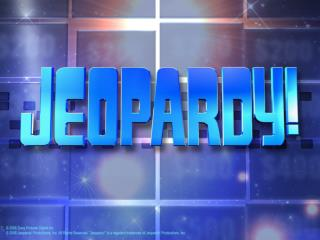DownloadDownload PresentationFinal Jeopardy

# Final Jeopardy

Download Presentation## Final Jeopardy

- - - - - - - - - - - - - - - - - - - - - - - - - - - E N D - - - - - - - - - - - - - - - - - - - - - - - - - - -
##### Presentation Transcript

1. Final Jeopardy Math!

2. Final Jeopardy 1x10-10 – water is neutral, therefore has pH of 7 1000=10x10x10=103 means change of 3 More basic so pH =7+3=10 pH 10 means [H+] = 1x10-10 A substance 1000x more basic than water would have what [H+] concentration?

3. \$200 What is a base? (Number 12 on review) This type of substance is generally slippery and releases OH- in solution.

4. \$400 What are acids? (Number 11) These substances taste sour.

5. \$600 What are bases? These substances generally taste bitter.

6. \$800 What are both acids and bases (Numbers 11 and 12 on review) These substances are good electrolytes.

7. \$1000 What are acids? These substances release H+ ions in solution.

8. \$200 What is weak? (Number 4) This type of acids and bases partially dissociate in water.

9. \$400 Mg(OH)2 + H2SO4 MgSO4 + H2O Base Acid Salt Water (Number 1) Identify the parts of the following neutralization reaction: Mg(OH)2 + H2SO4 MgSO4 + H2O

10. \$600 What is strong? This type of acid or base completely disassociates in water.

11. \$800 Water and a salt. These are the products when an acid and a base react together.

12. \$1000 What is double replacement? (Number 2) Neutralization reactions are this type of reaction.

13. \$200 What are bases? (Number 5) These substances turn red litmus paper blue.

14. \$400 What are acids (number 6)? These substances turn blue litmus paper red.

15. \$600 10 (Number 8) A solution with a pH of 14 is how many more times basic than a solution with a pH of 13?

16. \$800 A (Number 27) How should you make an acidic solution? a. add acid to water b. add water to acid c. add both at the same time d. you should never make an acidic solution because it’s too dangerous

17. \$1000 • Draw the pH scale. Label the following: • The range of pH’s shown on the scale • Where a neutral solution would be • Where the strongest acids and strongest bases would be

18. \$200 • base b) acid c) acid d) acid • Number 9 a-d • Identify each of the following as acids or bases: • NaOH • HCl • HF • HNO3

19. \$400 e) base f) base g) acid h) base (Number 9:e-g) • Identify each of the following as an acid or base: • Al(OH)3 • KOH • HI • NH4OH

20. \$600 Sour / high If you ever tasted HCl(which you wouldn’t because you are smart), it would most likely taste _____ and have a _____ concentration of H+ ions.

21. \$800 Slippery / low Calcium hydroxide feels _________ and has a _____ concentration of H+ ions.

22. \$1000 Al+3(OH)-1 Al(OH)3 What is the formula of Aluminum hydroxide?

23. \$200 pH = 6.44 pOH=14-6.44=7.56 What are the pH and pOH of a solution with a [H+] of 3.64 x 10-7?

24. \$400 0.26 mol/0.457 L = 0.57 M pOH =-log[0.57] = 0.24 What is the pOH of a solution in which 0.26 moles of sodium hydroxide (NaOH) was dissolved in .457 L of water.

25. \$600 1000 A solution with a pH of 4 is how many times more acidic than a solution with a pH of 7?

26. \$800 Number 13! Complete the following table:

27. \$1000 10000 = 10x10x10x10 = 104 Means a change in pH of 4, so it has pH of 5 What pH would a substance that is 10000x more acidic than one with a pH 9 have?

28. \$200 Strong Base (Number 18) If calcium hydroxide completely dissociates into positive and negative ions in water, then it is a _________.

29. \$400 Weak acid. (Number 19) If HC2H3O2 partially dissociates in water, then it is a _______________________.

30. \$600 True/False/False/True (Number 24) True or False? _____Strong acids almost completely dissociate in water while weak acids partially dissociate in water. _____Strong acids partially dissociate in water while weak acids almost completely dissociate in water. _____Weak acids always have a lower pH than strong acids. _____Strong acids produce hydroxide ions in solution and weak acids produce hydronium ions in solution.

31. \$800 10.3 M NaOH (Number 25) Which solution is more concentrated, 10.3 M NaOH or 7.5 M HCl?

32. \$1000 Strong – bright weak – dim, non – turned off (Number 26) What happens to a light bulb connected to an aqueous solution when you have a strong electrolyte? Weak electrolyte? Nonelectrolyte?